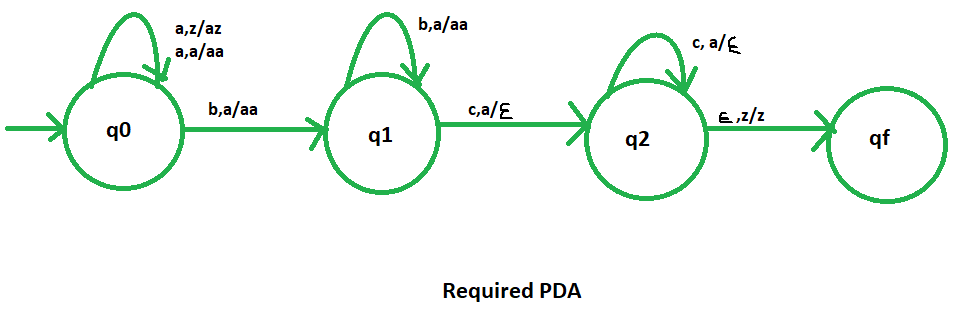Open In App
Related Articles
• Write an Interview Experience
• Automata Tutorial

# NPDA for accepting the language L = {ambnc(m+n) | m,n ≥ 1}

Prerequisite – Pushdown automata, Pushdown automata acceptance by final state

Problem – Design a non deterministic PDA for accepting the language L = {| m,n ≥ 1}, i.e.,

L = {abcc, aabccc, abbbcccc, aaabbccccc, ......}

In each of the string, the total sum of the number of ‘a’ and ‘b’ is equal to the number of c’s. And all c’s are come after ‘a’ and ‘b’.

Explanation – Here, we need to maintain the order of a’s, b’s and c’s.That is, all the a’s  are coming first and then all the b’s are coming after that all the c’s. Thus, we need a stack along with the state diagram. The count of a’s, b’s and c’s is maintained by the stack. We will take 2 stack alphabets:= { a, z }

Where,= set of all the stack alphabet z = stack start symbol

Approach used in the construction of PDA – As we want to design a NPDA, thus every time ‘a’ comes before ‘b’and ‘b’ comes before ‘c’. When ‘a’ comes then push it in stack and if again ‘a’ comes then also push it. After that, when ‘b’ comes then push ‘a’ into the stack and if again ‘b’ comes then also push it. And then when ‘c’ comes, pop one ‘a’ from the stack each time. So, at the end if the stack becomes empty then we can say that the string is accepted by the PDA.

Stack transition functions –(q0, a, z)(q0, az)(q0, a, a)(q0, aa)(q0, b, a)(q1, aa)(q1, b, a)(q1, aa)(q1, c, a)(q2,)(q2, c, a)(q2,)(q2,, z)(qf, z)

Where, q0 = Initial state qf = Final state= indicates pop operation